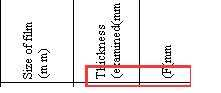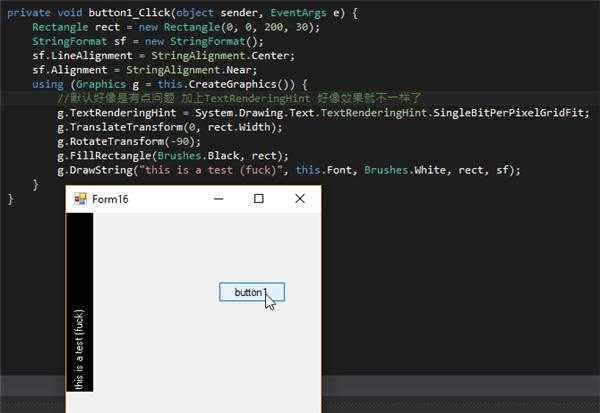﻿ Graphics画图转置 特殊字符位置跑到行前 | Code Bye

# Graphics画图转置 特殊字符位置跑到行前

4年前 (2016-09-17) 483次浏览
http://bbs.csdn.net/topics/39200948610

4030

```        void Form1_Paint(object sender, PaintEventArgs e)
{
var g = e.Graphics;
//g.TextRenderingHint = System.Drawing.Text.TextRenderingHint.SingleBitPerPixelGridFit;
using (var path = new GraphicsPath())
{
path.AddString("examined(mm)\r\nF(mm)", Font.FontFamily, 1, 24, new Point(10, 80), new StringFormat());
g.FillPath(Brushes.Blue, path);
path.Transform(GetMatrix(270, 10, 80, 0, 80));
g.FillPath(Brushes.Red, path);
}
}
Matrix GetMatrix(double angle, int a=0, int b=0, int mx = 0, int my = 0)
{
var n = angle / 180 * Math.PI;
var n1 = (float)Math.Cos(n);
var n2 = (float)Math.Sin(n);
var n3 = (float)-Math.Sin(n);
var n4 = (float)Math.Cos(n);
return new Matrix(n1, n2, n3, n4, a * (1 - n1) + b * n2 + mx, b * (1 - n4) + a * n3 + my);
}
```

CodeBye 版权所有丨如未注明 , 均为原创丨本网站采用BY-NC-SA协议进行授权 , 转载请注明Graphics画图转置 特殊字符位置跑到行前

[1034331897@qq.com]